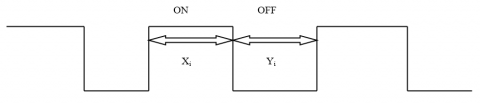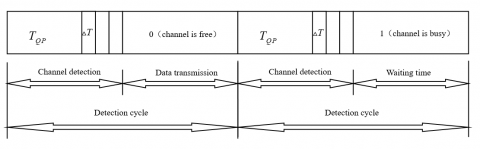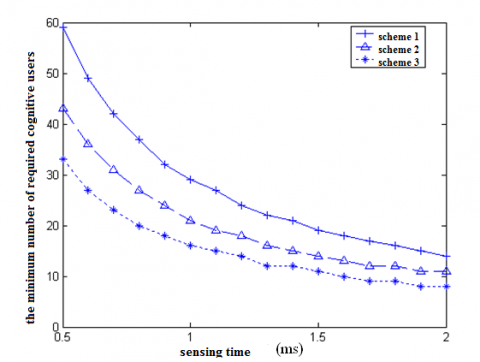# A Cooperative Spectrum Sensing Algorithm to Minimize the Sensing Overhead of Cognitive Radio System

A Cooperative Spectrum Sensing Algorithm to Minimize the Sensing Overhead of Cognitive Radio System

Dingxue Wu Wenping Fan Feng Xue Guosong Jiang

Computer Department, Huanggang Normal University, Huanggang, 438000, China

Radio Management Committee of Hubei, Wuhan 430080, China

Corresponding Author Email:
hustjgs@126.com
Page:
193-197
|
DOI:
https://doi.org/10.18280/ejee.210210
9 January 2019
|
Accepted:
20 March 2019
|
Published:
30 June 2019
| Citation

OPEN ACCESS

Abstract:

Cognitive radio (CR) technology is an emerging technology that overcomes the scarcity and poor utilization of spectrum resources. Under the constraint of system energy, this paper puts forward a cooperative spectrum sensing algorithm to minimize the sensing overhead, considering the mutual relation between sensing duration and the number of cognitive users. The simulation results show that, when the selected cognitive users face the same mean signal-to-noise ratio (SNR), the system overhead can be minimized by determining the proper number of required cognitive users and sensing duration. Thus, the proposed algorithm was proved valid.

Keywords:

1. Introduction

Fewer and fewer spectrum resources are available, due to the rapid growth of radio communication services. In such a dynamic environment, it is necessary to fully utilize the scattered available resources. Cognitive radio (CR) technology provides a desirable solution to the task .

The core of CR technology is spectrum sensing, which aims to effectively use spectrum hole without disturbing the existing primary users. In practice, however, primary users are disturbed inevitably, because cognitive users often need to distinguish primary users from weak receiving signals. After all, cognitive users neither have signaling interaction with primary users, nor know the geographic location of primary users. What is worse, cognitive users often cannot achieve the required detection performance, under the impacts of multipath fading and shadow effect.

The above problems of spectrum sensing can be solved in either space domain or time domain. In the space domain, more cognitive users should be involved in spectrum sensing. In the time domain, the sensing duration should be extended or the sampling rate should be enhanced. Despite enhancing sensing performance, these solutions may push up the sensing overhead. Considering the resource constraint of the CR system, it is imperative to achieve the required sensing performance with the minimal sensing overhead. The relevant studies are briefly reviewed below.

In the space domain, the sensing performance is affected by the growing correlation of the signals received indirectly by cognitive users, which are in cooperation with each other. To eliminate the correlation effect, Reference  proposes an algorithm that selects cognitive users by geographic location. Reference  determines the proper number of cognitive users to reduce the disturbance on primary users. Reference  chooses unrelated cognitive users in the light of their geographic location. Reference  sets up an algorithm to identify the minimum number of cognitive users that satisfy the required performance, but failing to consider the user difference in signal-to-noise ratio (SNR).

In the time domain, Reference  optimizes the sensing transmission strategy to maximize the throughput of cognitive users. Reference  presents optimal sensing parameters to control the overhead, considering the effect of SNR variation on cognitive users, but does not take account of how sensing duration affects the number of required cognitive users. Reference  optimizes the frame of spectrum sensing system under disturbance.

Under the constraint of system energy, this paper puts forward a cooperative spectrum sensing algorithm to minimize the sensing overhead, considering the mutual relation between sensing duration and the number of cognitive users. When the mean SNR is the same among all cognitive users, the proposed algorithm selects the users with relatively good performance, and determines the number of users and sensing duration that minimize the sensing overhead.

2. Modelling of Cooperative Spectrum Sensing System

Figure 1 shows the traditional cooperative spectrum sensing system. In this model, each cognitive user detects the signal from each primary user separately, and determines the appearance/absence of the primary user through soft- or hard-decision by certain fusion rules. Based on spectrum sensing, the hard-decision method is used by cognitive users. The cooperative spectrum sensing is implemented through the following steps.

Step 1. Each cognitive user completes spectrum sensing independently, and makes a binary decision on the occupation/openness of the spectrum.

Step 2. Each cognitive user transfers its decision to the base station through the corresponding control channel.

Step 3. The base station processes the decision by certain rules, generates the final decision of the system, and transfers the final decision to each cognitive user.

## 1.pngFigure 1. Model of cooperative spectrum sensing system

2.2 Spectrum utilization

As shown in Figure 2, each spectrum is either open or occupied. Then open state and occupied state are independent and distributed identically. In the open state, the primary users do not communicate, and the cognitive users can transfer data, without disturbing primary users. Once detecting primary user(s), cognitive users must cease to use the spectrum unconditionally. In the occupied state, the spectrum is loaded with the communication of primary users. The above utilization process forms a continuous Markov process.

## 2.pngFigure 2. The open state and occupied state of each spectrum

3. Cooperative Spectrum Sensing Algorithm for Minimal Overhead

3.1 Counting rule-based algorithm

This subsection formulizes the sensing performance of each cognitive user, and derives the formula of system performance based on counting rule when the cognitive users face different SNRs. Special attentions were paid to cases like AND and OR.

In the CR system, each cognitive user performs spectrum sensing. The operation of each user can be described as a binary hypothesis testing process:

\begin{align} & {{H}_{0}}:{{r}_{i}}={{n}_{i}} \\ & {{H}_{1}}:{{r}_{i}}={{h}_{i}}s+{{n}_{i}} \\\end{align}   (1)

The output statistic of spectrum sensing can be expressed as ${{Y}_{i}}={{\sum\limits_{m=0}^{M}{\left| {{r}_{i}}(m) \right|}}^{2}}$. Let τ be the judgement threshold. Then, the detection probability and false alarm probability of each user can be respectively expressed as:

${{p}_{d}}_{i}=\Pr ({{Y}_{i}}>\tau \left| {{H}_{1}} \right.)=Q\left( \frac{\tau -M\sigma _{n}^{2}(1+{{\gamma }_{i}})}{\sigma _{n}^{2}\sqrt{2M(1+2{{\gamma }_{i}})}} \right)$   (2)

${{p}_{fi}}=\Pr ({{Y}_{i}}>\tau \left| {{H}_{0}} \right.)=Q\left( \frac{\tau -M\sigma _{n}^{2}}{\sigma _{n}^{2}\sqrt{2M}} \right)$   (3)

3.2 System performance at different SNRs

Assuming that cognitive users adopts the hard-decision method, the base station merges the 1-bit decision of each user by the counting rule-based algorithm.

If the cognitive users face different SNRs, the formulas of the total detection probability and the total false alarm probability will become very complicated. For simplicity, each cognitive user was assumed as independent. According to the central-limit theorem, the detection probability and false alarm probability can be respectively depicted as:

${{P}_{f}}=Q(\frac{\alpha -\sum\limits_{i=1}^{N}{{{p}_{fi}}}}{\sqrt{\sum\limits_{i=1}^{N}{{{p}_{fi}}}-\sum\limits_{i=1}^{N}{{{({{p}_{fi}})}^{2}}}}})$   (4)

${{P}_{d}}=Q(\frac{\alpha -\sum\limits_{i=1}^{N}{{{p}_{di}}}}{\sqrt{\sum\limits_{i=1}^{N}{{{p}_{di}}-\sum\limits_{i=1}^{N}{{{({{p}_{di}})}^{2}}}}}})$   (5)

where N is the number of cognitive users. With the increase of N, the detection probability gradually approaches one, while the false alarm probability approximates zero.

The above analysis shows that, when all cognitive users face the same SNR, as assumed in the traditional algorithm, the detection probability increases towards one, with the growth in the number of users. Meanwhile, when the cognitive users face different SNRs, the detection probability declines with the increase in the number of users. In this case, the system performance cannot be optimized, even if all users participate in spectrum sensing.

When the cognitive users have different SNRs, the system performance can be assured by selecting the users with relatively good performance, and determining the proper sensing duration.

In the CR system, a high detection probability means small disturbance on primary users, while a low false alarm probability showcases improvement of spectrum efficiency. In this paper, the design principle of cooperative spectrum sensing algorithm is to minimize the overhead of system sensing without suppressing detection probability or pushing up false alarm probability. The spectrum sensing and data transfer procedure of cognitive users are illustrated in Figure 3 below.

## 3.pngFigure 3. The spectrum sensing and data transfer procedure of cognitive users

Considering its correlations with sensing duration and SNR, the number of cognitive users can be expressed as L(TQP,γ), with TQP being the system sensing duration and γ being the SNR of the received signal. Let Tsbe the sensing duration and ∆T be the time to transfer the sensed data to the base station. Then, the objective and constraints of spectrum sensing can be obtained as:

\begin{align} & \min L({{T}_{QP}},\gamma )({{T}_{QP}}+\vartriangle T) \\ & s.t.{{P}_{d}}(L)\ge {{P}_{D}} \\ & {{P}_{f}}(L)\le {{P}_{F}} \\\end{align}    (6)

where PD and PF are the detection probability and the false alarm probability satisfying the required system performance, respectively.

From the constraint conditions, it can be seen that the system head growth rate is proportional to the number of cognitive users involved in cooperative sensing. Therefore, the number of cognitive users should be minimized, while satisfying the required system performance.

Let L(TQP,γ) be the minimum number of cognitive users under the above constraints. Then, the value of L(TQP,γ) (simply denoted as L) can be obtained as:

$L=\left\lceil {{\left[ \frac{{{Q}^{-1}}({{P}_{D}})\sqrt{\overline{{{\sigma }^{2}}}}-{{Q}^{-1}}({{P}_{F}})\sqrt{{{p}_{f}}(1-{{p}_{f}})}}{{{\overline{P}}_{d}}-{{p}_{f}}} \right]}^{2}} \right\rceil$  (7)

The expression can be further simplified as L=max(L1,L2), with L1 and L2 being the minimum number of cognitive users to satisfy required false alarm probability and that to satisfy required detection probability, respectively. The values of L1 and L2 can be respectively computed by:

${{L}_{1}}=\left\lceil {{\left[ \frac{{{Q}^{-1}}({{P}_{F}})\sqrt{{{p}_{f}}(1-{{p}_{f}})}}{\lambda -{{p}_{f}}} \right]}^{2}} \right\rceil$    (8)

${{L}_{2}}=\left\lceil {{\left[ \frac{{{Q}^{-1}}({{P}_{D}})\sqrt{\overline{{{\sigma }^{2}}}}}{\lambda -{{\overline{P}}_{d}}} \right]}^{2}} \right\rceil$    (9)

If proper parameters are selected, L2 can be generally taken as the minimum number of cognitive users to achieve the required system performance. Formula (9) shows that the required number of cognitive users is negatively correlated with the SNR faced by each user. The SNR value was fixed to determine the maximum number of cognitive users:

$L={{\left\lceil \frac{{{Q}^{-1}}({{P}_{D}}){{p}_{d}}(1-{{p}_{d}})}{\lambda -{{p}_{d}}} \right\rceil }^{2}}$     (10)

where pd is sensing duration. Taking the derivative of system sensing duration TQP, the following formula can be derived:

$\frac{\partial F}{\partial {{T}_{QP}}}=\frac{16(\lambda -{{p}_{d}})\left[ 2({{T}_{QP}}+\vartriangle T)(-\frac{\partial {{p}_{d}}}{\partial {{T}_{QP}}})-(\lambda -{{p}_{d}}) \right]}{{{Q}^{-1}}{{({{P}_{D}})}^{2}}{{({{T}_{QP}}+\vartriangle T)}^{2}}}$   (11)

Since $\frac{\partial {{p}_{d}}}{\partial {{T}_{QP}}}>\text{0}$, the derivative can be zero with proper parameters.

To sum up, the system overhead of an energy-constrained spectrum sensing system can be minimized with proper number of cognitive users and sensing duration. The proposed algorithm is then verified through computer simulation.

4. Performance Verification

Before simulation, it is assumed that cognitive users are randomly distributed in a 30km-radius circle, with the base station being the center; the mean SNR faced by cognition users was -20dB; the base station knew the SNR and geographic location of each user. On this basis, three SNR schemes with the same mean SNR were simulated. From scheme 1 to scheme 3, the variance increased gradually.

\begin{align} & Scheme1:SNR(i)=\overline{SNR}; \\ & Scheme2:SNR(i)=SN{{R}_{\max }}-(SN{{R}_{\max }}-\overline{SNR})\frac{2i}{N-1}; \\ & Scheme3:SNR(i)=SN{{R}_{\max }}-(SN{{R}_{\max }}-\overline{SNR})\frac{6{{i}^{2}}}{(N-1)(2N-1)}; \\\end{align}  (12)

Through the simulation, the relationship between sensing duration and the minimum number of required cognitive users, and that between sensing duration and sensing overhead are respectively plotted as Figure 4 and Figure 5, where the minimum sensing overhead is marked by an arrow and the corresponding scheme by a label.

## 4.pngFigure 4. The relationship between sensing duration and the minimum number of required cognitive users

## 5.pngFigure 5. The relationship between sensing duration and sensing overhead

As shown in the figures, the system detection probability increased, while the false alarm probability and the number of required cognitive users declined, with the growth in sensing duration. Taking scheme 2 for instance, the minimum number of required cognitive users was 15, and the optimal sensing duration was about 1.7ms. The simulation verifies the correctness and the efficiency of the proposed algorithm.

5. Conclusions

Our research reveals that the system sensing performance cannot be optimal even if all cognitive users participate in spectrum sensing, when the spectrum resources are limited. Therefore, the author selected the users with relatively good performance for spectrum sensing. The simulation results show that, when the selected cognitive users face the same mean SNR, the system overhead can be minimized by determining the proper number of required cognitive users and sensing duration.

Acknowledgment

This work is supported by Hubei Provincial Natural Science Foundation of China (2014CFC1099), Excellent Young and Middle-aged Science and Technology Innovative Research Team in Higher Education Institutions of Hubei Province (Key Technology and Application of Big Data), Thanks to the reviewers for the valuable comments helping to improve the quality of the manuscript.

References

 Mitola, J., Maquire, G.Q., Jr. (1999). Cognitive radio: Making software radios more personal. IEEE Personal Communications, 6(4): 13-18. https://doi.org/10.1109/98.788210

 Kim, H., Shin, K.G. (2008). In-band spectrum sensing in cognitive radio networks. Energy Detection or Feature Detection. in: Proceedings of the 14th Annual International Conference on Mobile Computing and Networking, pp. 14-25. https://doi.org/10.1145/1409944.1409948

 Cabric, D., Mishra, S.M., Brodersen, R.W. (2004). Implementation issues in spectrum sensing for cognitive radios.in: Proceedings of 2004 Conference Record the Thirty-Eighth Asilomar Conference on Signals, Systems and Computers, pp. 772-776. https://doi.org/10.1109/ACSSC.2004.1399240

 Selen, Y., Tullberg, H., Kronander, J. (2008). Sensor selection for cooperative spectrum sensing. Proceedings of 2008 3rd IEEE Symposium on New Frontiers in Dynamic Spectrum Access Networks. Chicago, IL, pp. 1-11. https://doi.org/10.1109/DYSPAN.2008.51

 Zhang, W., Mallik, R.K., Letaief, K.B. (2008). Cooperative spectrum sensing optimization in cognitive radio networks. in: Proceedings of 2008 IEEE International Conference on Communications. Beijing, China, pp. 3411-3415. https://doi.org/10.1109/ICC.2008.641

 Huang, S.H., Liu, X., Ding, Z. (2009). Optimal sensing-transmission structure for dynamic spectrum access. Proceedings of 2009 IEEE on INFOCOM. Rio de Janeiro, pp. 2295-2303. https://doi.org/10.1109/INFCOM.2009.5062155

 Min, A.W., Shin, K.G. (2009). An optimal sensing framework based on spatial RSS-profile in cognitive radio networks. Proceedings of the 2009 6th Annual IEEE communications society conference on Sensor, Mesh and Ad Hoc Communications and Networks. Rome, pp. 1-9. https://doi.org/10.1109/SAHCN.2009.5168908

 Lee, W.Y., Akyildiz, I.F. (2008). Optimal spectrum sensing framework for cognitive radio networks. IEEE Transactions on Wireless Communications, 2008, 7(10): 3845-3857. https://doi.org/10.1109/T-WC.2008.070391

 Darsena, D., Gelli, G., Verde, F. (2017). An opportunistic spectrum access scheme for multicarrier cognitive sensor networks. IEEE Sensors Journal, 17(8): 2596–2606. https://doi.org/10.1109/JSEN.2017.2674181

 Shah, H.A. Koo, I. (2018). A novel physical layer security scheme in OFDM-based cognitive radio networks. IEEE Access, 6: 29486–29498. https://doi.org/10.1109/ACCESS.2018.2842826

 Khoshkholgh, M.G., Yamchi, N.M., Navaie, K., Yanikomeroglu, H., Leung, V.C.M., Shin, K.G. (2015). Radio resource allocation for OFDM-based dynamic spectrum sharing: Duality gap and time averaging. IEEE Journal on Selected Areas in Communications, 33(5): 848–864. https://doi.org/10.1109/JSAC.2014.2361080

 Chami, M., Pischella, M., Le Ruyet, D. (2017). Resource allocation for OFDM-based multiuser cooperative underlay cognitive systems. EURASIP Journal on Wireless Communications and Networking, (1): 180.

 Sabuj, S.R., Hamamura, M. (2017). Uplink modeling of cognitive radio network using stochastic geometry. Performance Evaluation, 117: 1–15. https://doi.org/10.1016/j.peva.2017.09.001

 Miyoshi, N., Shirai, T. (2014). Cellular networks with α-Ginibre configurated base stations. The Impact of Applications on Mathematics, Springer, New York. https://doi.org/10.1007/978-4-431-54907-9_16

 Deng, N., Zhou, W., Haenggi, M. (2015). The Ginibre point process as a model for wireless networks with repulsion. IEEE Transactions on Wireless Communications, 14(1): 107–121. https://doi.org/10.1109/TWC.2014.2332335

 Nakata, I., Miyoshi, N. (2014). Spatial stochastic models for analysis of heterogeneous cellular networks with repulsively deployed base stations. P Performance Evaluation, 78: 7-17. https://doi.org/10.1016/j.peva.2014.05.002

 Haenggi, M. (2012). Stochastic Geometry for Wireless Networks. Cambridge University. Press, Cambridge. https://doi.org/10.1017/CBO9781139043816.001

 ElSawy, H., Hossain, E. (2014). On stochastic geometry modeling of cellular uplink transmission with truncated channel inversion power control. IEEE Transactions on Wireless Communications, 13(8): 4454–4469. https://doi.org/10.1109/TWC.2014.2316519

 ElSawy, H., Sultan-Salem, A., Alouini, M.S., Win, M.Z. (2017). Modeling and analysis of cellular networks using stochastic geometry: A tutorial. IEEE Communications Surveys & Tutorials, 19(1): 167–203. https://doi.org/10.1109/COMST.2016.2624939

 Novlan, T.D., Dhillon, H.S., Andrews, J.G. (2013). Analytical modeling of uplink cellular networks. IEEE Transactions on Wireless Communications, 12(6): 2669–2679. https://doi.org/10.1109/TWC.2013.050613.120325

 Lee, C.H., Haenggi, M. (2012). Interference and outage in Poisson cognitive networks. IEEE Transactions on Wireless Communications, 11(4): 1392–1401. https://doi.org/10.1109/TWC.2012.021512.110131

 Guo, Y.C., Niu, K., Lin, J.R. (2013). Stochastic geometric analysis of the uplink throughput in cognitive radio cellular networks. China Communications, 10(8): 44–53. https://doi.org/10.1109/CC.2013.6633744